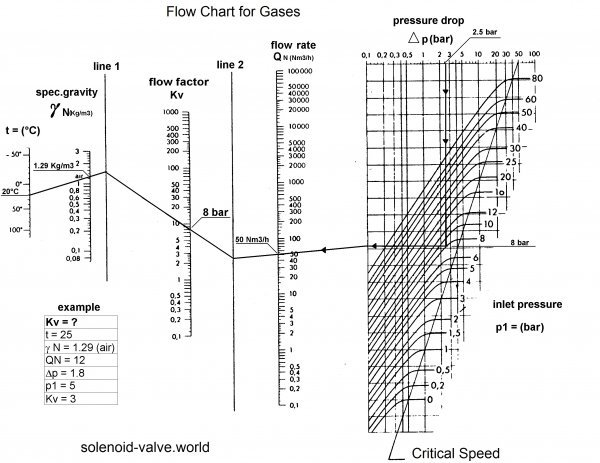# Calculating Flow Rates and Pressure Drops.

Calculating the pressure drop or flow rate of media through a solenoid valve can be quite a head ache, especially when the media is a compressible gas. Design and Process Engineers will know the daunting task ahead when calculating pressure drop or flow. Typically an internet search will provide a website or technical knowledge data base that can provide some answers, but with no way to verify the results leading to concerns on accuracy and costly mistakes.

Here we offer simplified flow charts to calculate the flow or pressure drop of both liquids and gases through a solenoid valve.

## Flow chart for liquids.

Typically flow (m3hr) = Cv flow factor x multiplied by square root of (pressure drop divided by fluid density). Density of water is 1, diesel fuel 0.9.### How to calculate flow for liquids:

Example n°1

How to calculate the flow coefficient (Kv) of a solenoid valve to obtain a water flow of 100 l/min with 5 bar pressure drop. Specific gravity of water=1kg/dm3

Draw a straight line intersecting the values 5 and 100 on the axis of pressure drop and flow rate.
Extend this line up to the mark line.
Draw a straight line intersecting value 1 on the axis of specific gravity up to the break point of the first straight line with the mark line.
This line intersects the Kv axis and gives you the value you were looking for : 45 l/min

Example n°2
How to calculate the flow coefficient (Kv) of a solenoid valve to obtain a water flow of 20 l/min with 4 bar pressure drop.
Specific gravity of water=1kg/dm3

-Draw a straight line intersecting the values 1 and 20 on the axis of specific gravity and Kv.
-Extend this line up to the mark line.
-Draw a straight line intersecting the value 4 on the axis of pressure drop and the break point of the first straight line with the mark line.
-This line intersects the axis of flow rate and gives you the value you were looking for : 40 l/min

## Flow chart for Gases.How to calculate flow for gases
Example: How to calculate the flow coefficient (Kv) of a solenoid valve to obtain an air flow at 20°C of 50 Nm3/h with 7 bar pressure (i.e 8 bar relative pressure), a pressure drop (delta p) of 2.5 bar, and a specific gravity of 1.29.

Draw a vertical straight line from the value 2.5 on the ?p axis down to the pressure curve (p1) of 8 bar.

Then horizontally transfer this break point to the vertical line of delta p= 0.1.
Draw a straight line from this new break point up to the point 50 on the flow rate axis, and extend it up to the mark line n°2.
Draw a straight line intersecting the values 20 and 1.29 on the axis of temperature and specific gravity.

Extend this line up to the mark line n°1.
Draw a straight line between the break points of mark lines n°1 and 2.

This line intersects the Kv axis and gives you the value you were looking for : 8l/mn